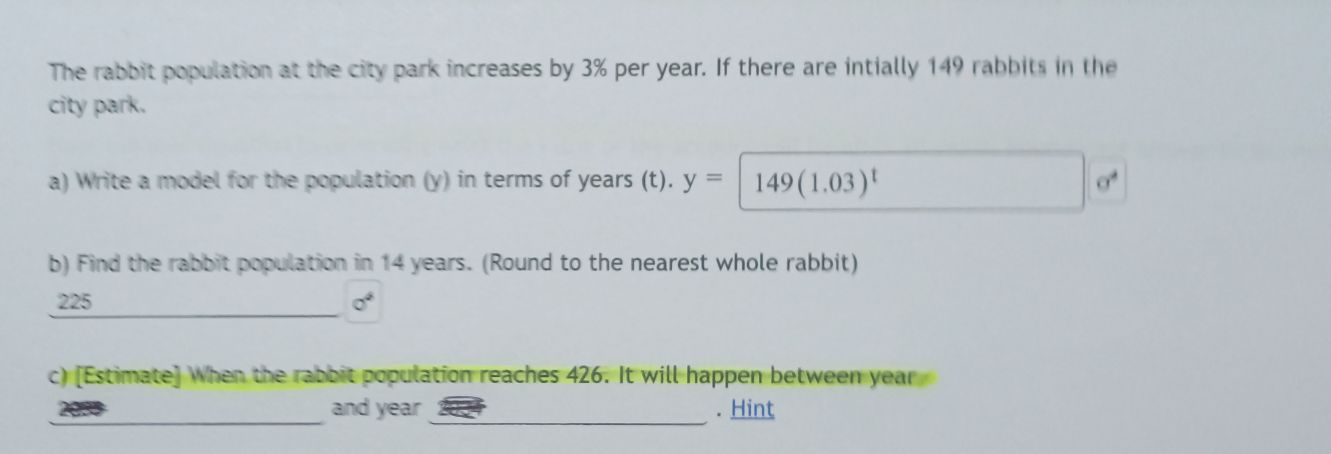### Still have math questions?

Algebra
QuestionThe rabbit population at the city park increases by $$3 \%$$ per year. If there are intially $$149$$ rabbits in the city park. a) Write a model for the population $$( y )$$ in terms of years $$( t ) . y = 149 ( 1.03 ) ^ { t } )$$

b) Find the rabbit population in $$14$$ years. (Round to the nearest whole rabbit)

$$225$$ c) [Estimate] When the rabbit population reaches 426. It will happen between year zes and year